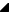Properties

Name

DescriptionReference

Represents property Reference.Units

Represents property Units.X1

X1,   Y1, X2 and   Y2 define a gradient vector for the linear gradient. This gradient vector provides starting and ending points onto which the gradient stops are mapped. The values of X1, Y1, X2 and Y2 can be either numbers or percentages.X2

X1,   Y1, X2 and   Y2 define a gradient vector for the linear gradient. This gradient vector provides starting and ending points onto which the gradient stops are mapped. The values of X1, Y1, X2 and Y2 can be either numbers or percentages.Y1

X1,   Y1, X2 and   Y2 define a gradient vector for the linear gradient. This gradient vector provides starting and ending points onto which the gradient stops are mapped. The values of X1, Y1, X2 and Y2 can be either numbers or percentages.Y2

X1,   Y1, X2 and   Y2 define a gradient vector for the linear gradient. This gradient vector provides starting and ending points onto which the gradient stops are mapped. The values of X1, Y1, X2 and Y2 can be either numbers or percentages.

Top

RiverSoftAVG SVG Component Library (RSCL) © 2013-2015, Thomas G. Grubb